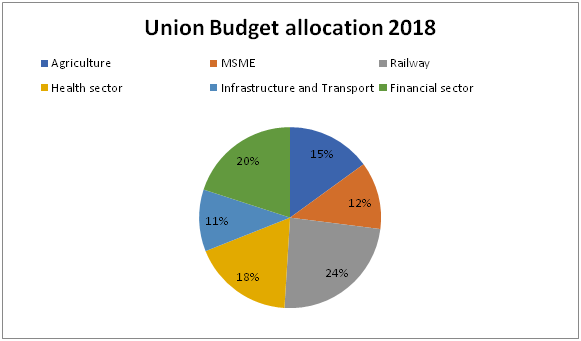# IBPS Clerk Prelims Quantitative Aptitude Questions 2019 (Day-16)

Dear Aspirants, Our IBPS Guide team is providing new series of Quantitative Aptitude Questions for IBPS Clerk Prelims 2019 so the aspirants can practice it on a daily basis. These questions are framed by our skilled experts after understanding your needs thoroughly. Aspirants can practice these new series questions daily to familiarize with the exact exam pattern and make your preparation effective.

[WpProQuiz 7401]

Application sums

1) A, B and C started the business by investing in the ratio of 3 : 6 : 5. 30 % of the profit goes to charity; the remaining will be shared by three of them. The share of B is Rs. 24000. Find the total profit?

a) Rs. 92000

b) Rs. 73000

c) 80000

d) Rs. 104000

e) None of these

2) 4 women and 5 men can do a work in 8 days. 8 women and 5 men can do a same work in 6 days. How long will 8 women and 5 men can take to do the work?

a) 6 days

b) 5 days

c) 8 days

d) 7 days

e) None of these

3) Applied to a bill for Rs. 50000. Find the difference between a discount of 25 % to that of two successive discounts of 20 % and 10 %?

a) Rs. 2250

b) Rs. 1500

c) Rs. 1750

d) Rs. 1800

e) None of these

4) A bag contains 5 red marbles, 6 yellow marbles and 4 green marbles. If three marbles are taken at randomly, then find the probability that 2 marbles are yellow?

a) 35/72

b) 53/78

c) 11/45

d) 27/91

e) None of these

5) Three-seventh of a number is equal to five-eight of another number. The difference of these two numbers is 22. Find the numbers?

a) 70 and 48

b) 52 and 30

c) 66 and 44

d) 85 and 63

e) None of these

Data interpretation

Directions (6 – 10) Study the following information carefully and answer the given questions:

The following pie chart shows the percentage distribution of union budget (In Rs.) allocated for various schemes in 2018.

Total Revenue = 90000 Crore6) Find the average amount allocated for Agriculture, Railway and Health sector together?

a) 18500 Crore

b) 17100 crore

c) 16800 Crore

d) 15700 Crore

e) None of these

7) Find the difference between the total amount allocated for MSME sector to that of Finance sector?

a) 6800 Crore

b) 5600 Crore

c) 7500 Crore

d) 7200 Crore

e) None of these

8) Find the ratio between the total amount allocated for Agriculture and Infrastructure & transport together to that of total amount allocated for Health and Finance sector together?

a) 13: 19

b) 5: 8

c) 11: 17

d) 22: 29

e) None of these

9) Total amount allocated for MSME is approximately what percentage more/less than the total amount allocated for Health sector?

a) 15 % more

b) 20 % less

c) 33 % less

d) 20 % more

e) 33 % more

10) In agriculture sector, the ratio between the total amount allocated to different categories namely, agriculture marketing infrastructure, Food processing, Fisheries and Aquaculture and other sources related to agriculture is 8 : 11 : 5 : 3, then find the difference between the total amount allocated to Food processing to that of Fisheries and Aquaculture?

a) 3400 Crore

b) 3000 Crore

c) 2800 Crore

d) 2500 Crore

e) None of these

Directions (1-5) :

Investment ratio = 3 : 6 : 5

B’s share = Rs. 24000

6’s = 24000

1’s = 4000

14’s = 4000*14 = 56000

70 % of total profit = 56000

(70/100)*total profit = 56000

Total profit = 56000*(100/70) = Rs. 80000

Total work = (men (or) women)*days

Work equal, so,

(4 w + 5 m)*8 = (8 w + 5 m)*6

32 w + 40 m = 48 w + 30 m

16 w = 10 m

8 w = 5 m = > 1 w = 5/8 m

4 w + 5 m = 4*(5/8) m+ 5 m = 5/2 m + 5 m = 15/2 m

8 w + 5 m = 5 m + 5 m = 10 m

Men            days

15/2            8

10               ?

(15/2)*8 = 10*x

X= (15/2)*(8/10) = 6 days

50000*(75/100) = Rs. 37500

50000*(80/100)*(90/100) = Rs. 36000

Required difference

= > 37500 – 36000

= > Rs. 1500

Total probability n(S) = 15C3

Required probability n(E) = 6C2 and 9C1

P(E) = n(E)/n(S) = 6C2 and 9C1 / 15C3

= > 27/91

(3/7) of a number = (5/8) of another number

(3/7)*x = (5/8)*y

(x/y) = (5/8)*(7/3) = 35/24

X : y = 35 : 24

11’s = 22

1’s = 2

The numbers are = 35*2 and 24*2 = 70 and 48

Directions (6-10) :

The average amount allocated for Agriculture, Railway and Health sector together

= > [(15 + 24 + 18)/100]*90000*(1/3)

= > (57/100)*30000 = 17100 crore

The total amount allocated for MSME sector

= > 90000*(12/100)

The total amount allocated for Finance sector

= > 90000*(20/100)

Required difference = 90000*[(20 – 12)/100] = 90000*(8/100) = 7200 Crore

The total amount allocated for Agriculture and Infrastructure & transport together

= > 90000*(26/100)

The total amount allocated for Health and Finance sector together

= > 90000*(38/100)

Required ratio = [90000*(26/100)] : [90000*(38/100)] = 26 : 38 = 13 : 19

Total amount allocated for MSME

= > 90000*(12/100) = 10800 Crore

Total amount allocated for Health sector

= > 90000*(18/100) = 16200 Crore

Required % = [(16200 – 10800)/16200]*100 = 33.33 % less

The ratio between the total amount allocated to different categories namely, agriculture marketing infrastructure, Food processing, Fisheries and Aquaculture and other sources related to agriculture

= >8 : 11 : 5 : 3

The difference between the total amount allocated to Food processing to that of Fisheries and Aquaculture

= > 90000*(15/100)*(6/27)

= > 3000 Crore